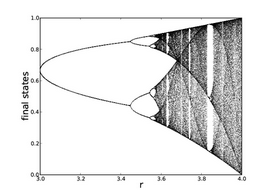# Complexity Explorer Santa Fe Institute

This course is no longer in session.## Introduction to Dynamical Systems and Chaos (Fall 2018)

• Welcome and Course Overview
• How to Take this Course
• Introduction to Functions
• Iteration
• Fixed Points and Stability
• Summary
• Homework (Optional)
• Homework Solutions (Optional)
• Test
• Introduction
• The Idea of the Derivative
• Differential Equations
• Computational Solutions
• Qualitative Solutions
• Summary
• Newton, Laplace, and Determinism
• Homework (Optional)
• Test
• Introducing the Logistic Equation
• Iterating the Logistic Equation
• The Butterfly Effect
• Randomness?
• Modeling Styles
• Lyapunov Exponents (Optional)
• Logistic Equation Programs
• Summary
• Homework (Optional)
• Test
• The Logistic Differential Equation
• Logistic Equation with Harvest
• Bifurcation Diagrams
• Bifurcations
• Hysteresis (Optional)
• Summary
• Homework (Optional)
• Test
• Final State Diagrams
• The Bifurcation Diagram
• Bifurcation Diagram Explorations
• Logistic Equation Programs
• Summary
• Homework (Optional)
• Test
• Introduction
• Universality in Maps
• Universality in Physical Systems
• Renormalization
• Summary
• Bifurcation Diagram Programs
• Homework (Optional)
• Test
• Lotka Volterra Differential Equations
• The Phase Plane
• The Hénon Map
• The Lorenz Equations
• Summary
• Hénon Map Program
• Homework (Optional)
• Test
• The Hénon Attractor
• The Lorenz Attractor
• Stretching and Folding
• Summary
• Homework (Optional)
• Test
• Diffusion
• Reaction-Diffusion Equations
• Computer Experiments
• Real Experiments
• Summary
• No Homework for Unit 9
• No Test for Unit 9
• Summary and Overview
• Interview: Stephen H. Kellert
• Interview: Stephen W. Morris
• Themes and Conclusions
• Farewell

#### Quiz scores are NOT recorded.

• You may come back to quizzes and take them as many times as you like
• When you are finished, clicking the "Score" button at the bottom of the test will show you the correct responses.

#### InstructionsThe bifurcation diagram for the logistic equation is shown above. Use this bifurcation diagram to determine the behavior of the logistic equation for the follow r values.